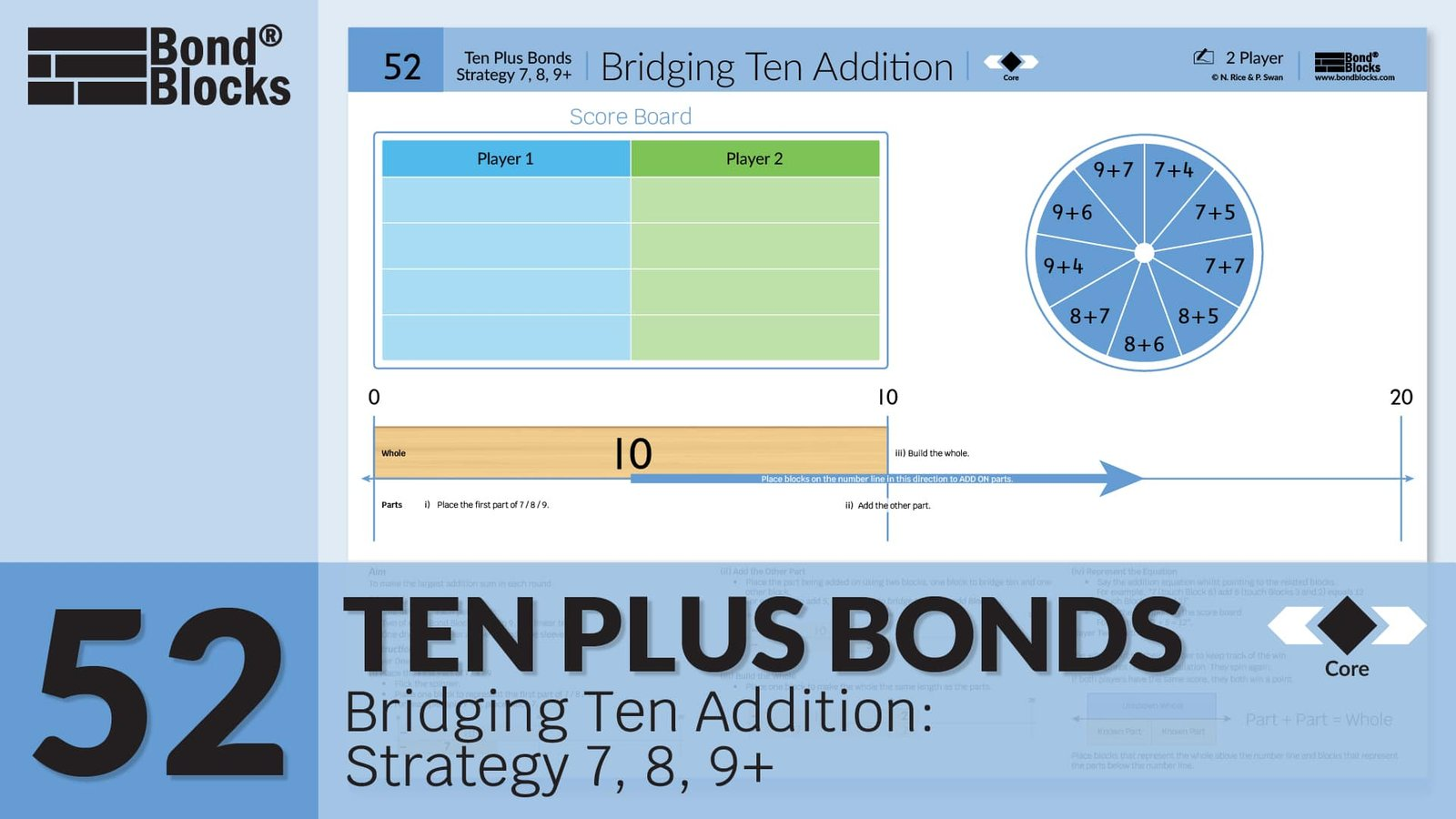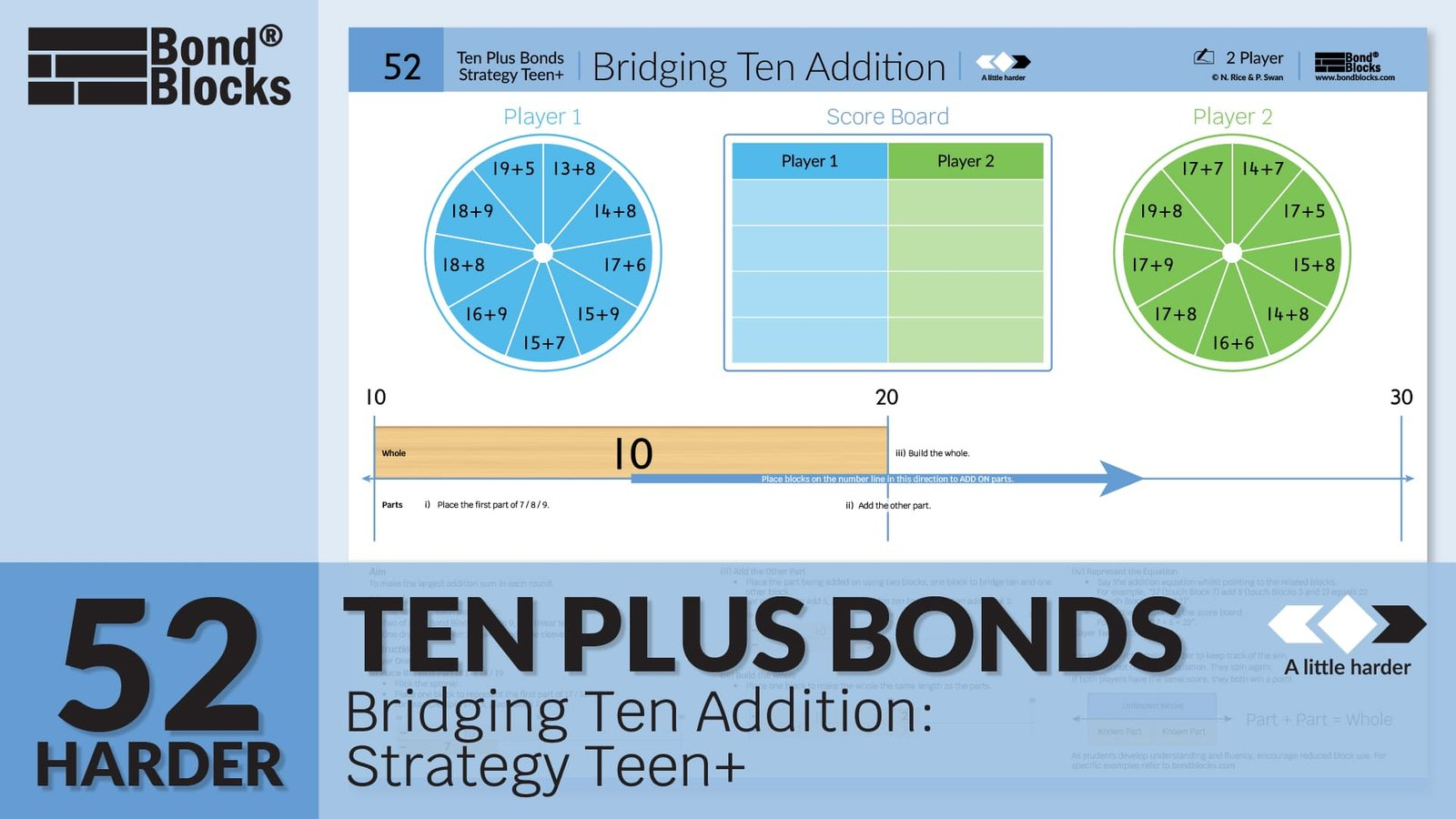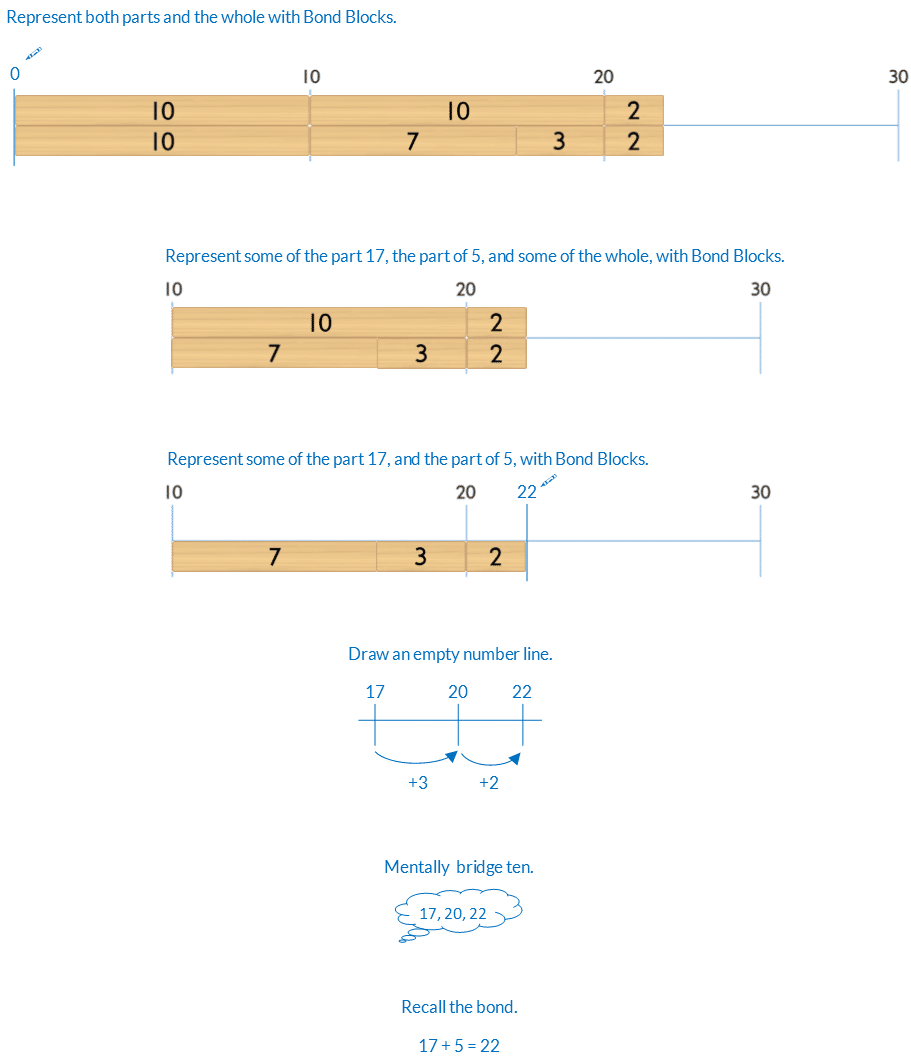Ten Plus Bonds: Strategy 7, 8, 9+### Mathematics

Develop the addition strategy of bridging ten, from a part of seven, eight, or nine for wholes up to 20.

For example, 7 + 5Prior knowledge of part-part-whole, bonds of 10 and place value partitioning using ten plus bonds is needed.

### Language

• bridge ten
• equals
• sum: the total resulting from addition## Differentiation

### A little easier

##### Counting order

Calculate each question in counting order, working clockwise around the spinner, from the 12 o’clock position. Do not flick the spinner. Students complete each type of equation:

• 7+
• 8+
• 9+

Assist students to identify patterns of adding on 3, 2 or 1 depending on the part being added on to.

##### Use Bond Blocks

If students have difficulty partitioning the second part, into one plus another part, use an additional block.

For example, to model 7 + 5Some students find it conceptually easier to first place the 7 and 5 blocks. Then place the 3 and 2 on top of the 5 block.

• From a top view they can see the bridging ten partition, 7 + 3 + 2.
• From a front view they can see the parts of 7 and 5.### A little harder

##### Bridging ten addition strategy teen +

Play “Ten Plus Bonds: Bridging Ten Addition Strategy Teen + a little harder”. In this game students have to bridge ten, to twenty, for a range of parts, for wholes up to 30.##### Reduce scaffolds

The blocks are a scaffold to support calculation. If students can calculate without some or all of the blocks encourage this. Below is a progression of reducing scaffolding, from most to least support, using bridging ten for 17 + 5.### Progression

In the next activity students apply bridging 10 to subtraction. Go to

##### Activity 53

Ten Plus Bonds: Bridging Ten Subtraction, Strategy Taking Away